# Team:NKU China/Model

Mathematical Model
AI-2 Supplier
Ordinary differential equations used to model the production of AI-2 in AI-2 controller.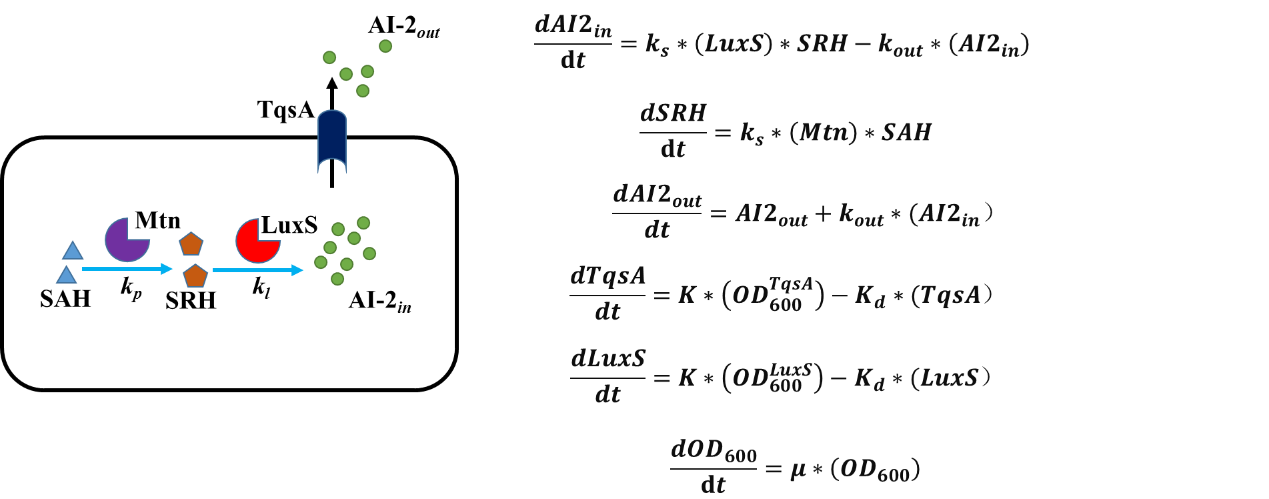Fig. 1: Ordinary differential equations used to model the production of AI-2 in AI-2 Suppliers.
AI-2 Consumer
Ordinary differential equations used to model the uptake of exogenously added AI-2 in AI-2 Consumers.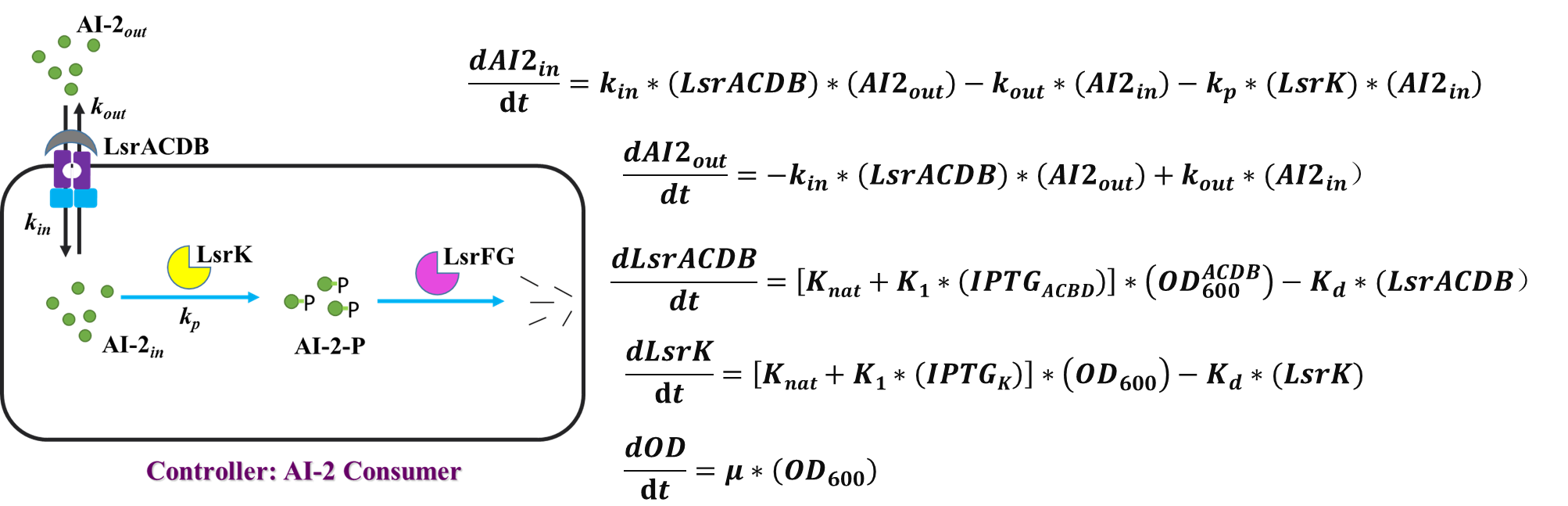Fig. 2: Ordinary differential equations used to model the uptake of exogenously added AI-2 in AI-2 Consumers.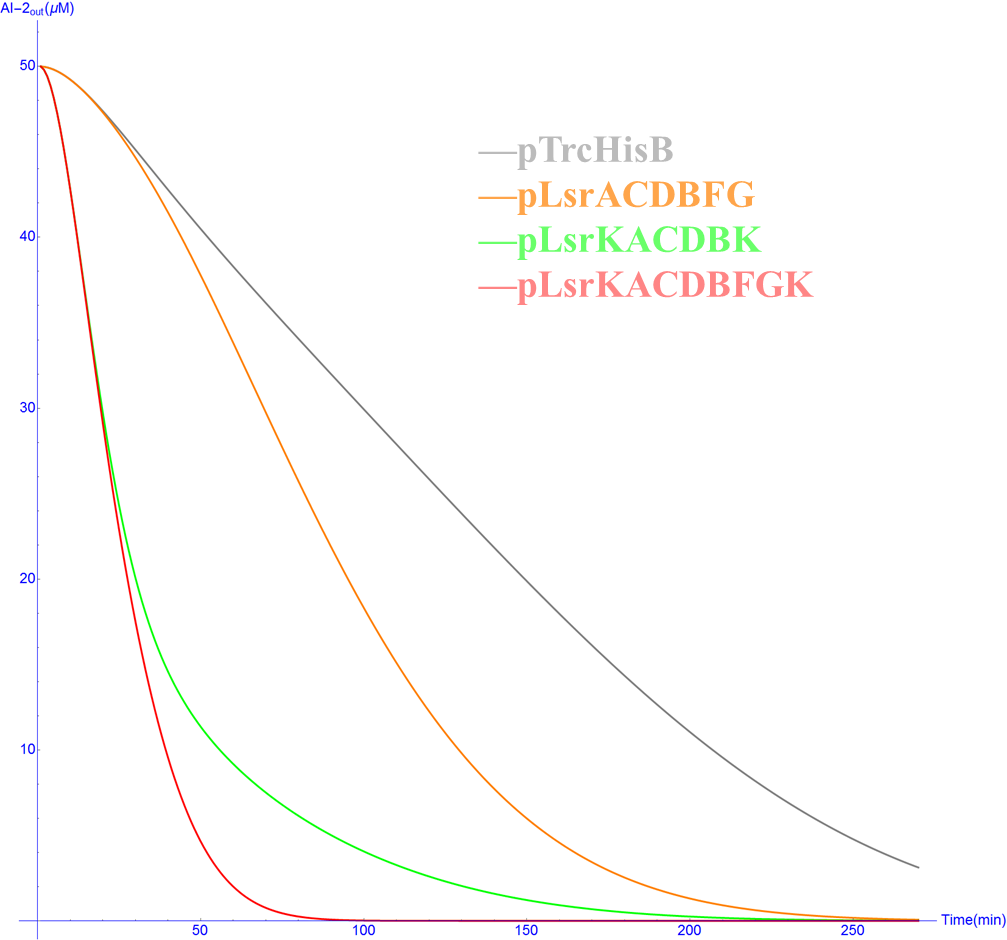Fig. 3: Simulation of AI-2 Consumer Devices: pTrcHisB, pLsrACDBFG, pLsrACDBK, pLsrACDBFGK
AI-2 Response Device
Ordinary differential equations used to model GFP expression of AI-2 Response Device.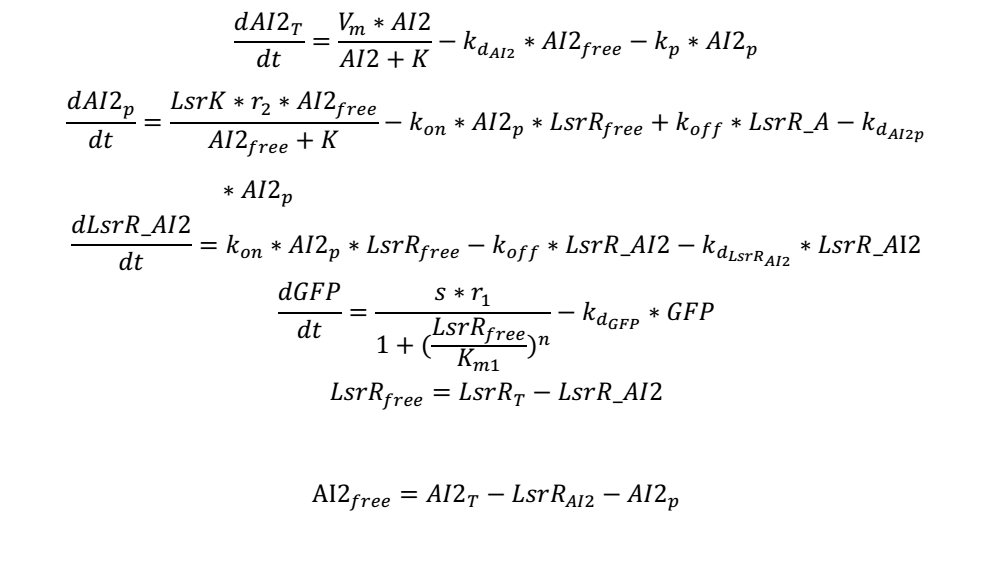Fig. 4: Ordinary differential equations used to model GFP expression of AI-2 Response Device.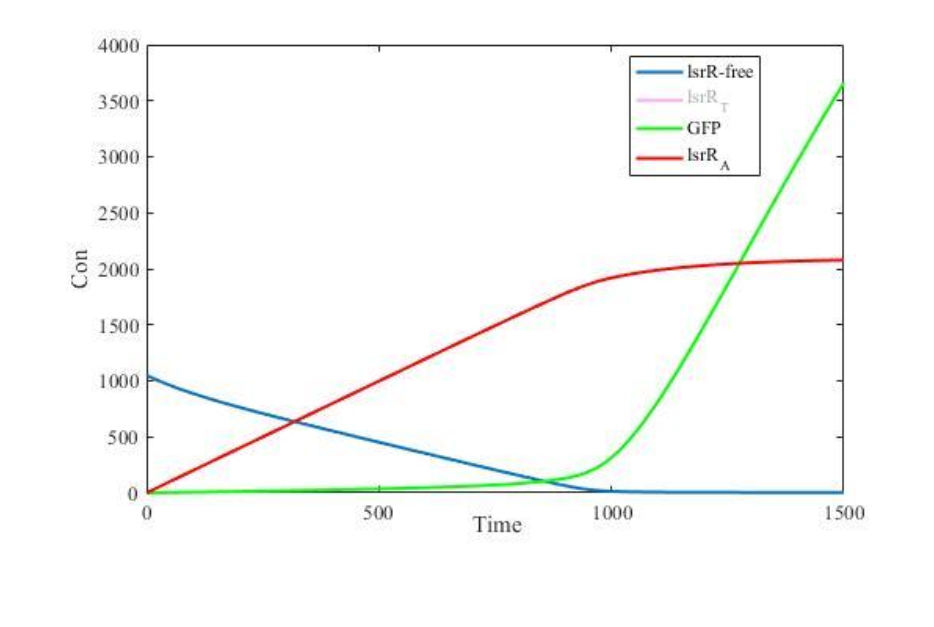Fig. 5: Simulation of GFP expression in AI-2 Response Devices